# Detect the Last item in a List using a for loop in PythonLast updated: Feb 20, 2023
4 min## #Detect the last item in a list using a for loop in Python

To detect the last item in a list using a `for` loop:

1. Use the `enumerate` function to get tuples of the index and the item.
2. Use a `for` loop to iterate over the `enumerate` object.
3. If the current index is equal to the list's length minus `1`, then it's the last item in the list.
main.py
```Copied!```my_list = ['one', 'two', 'three', 'four']

for index, item in enumerate(my_list):
if index != len(my_list) - 1:
print(item, 'is NOT last in the list ✅')
else:
print(item, 'is last in the list ❌')
``````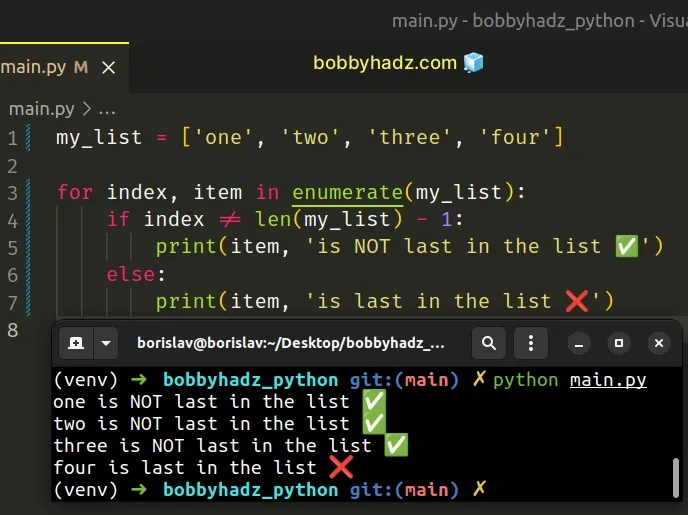We used the `enumerate()` function to get an `enumerate` object we can iterate over.

The enumerate function takes an iterable and returns an enumerate object containing tuples where the first element is the index and the second is the item.

main.py
```Copied!```my_list = ['one', 'two', 'three', 'four']

# 👇️ [(0, 'one'), (1, 'two'), (2, 'three'), (3, 'four')]
print(list(enumerate(my_list)))
``````

We used a `for` loop to iterate over the `enumerate` object and on each iteration, we check if the current index is NOT equal to the last index in the list.

## #Checking if the current index is equal to the last index

If the current index is not equal to the last index in the list, then the element is not the last list item.

main.py
```Copied!```my_list = ['one', 'two', 'three', 'four']

for index, item in enumerate(my_list):
if index != len(my_list) - 1:
print(item, 'is NOT last in the list ✅')
else:
print(item, 'is last in the list ❌')
``````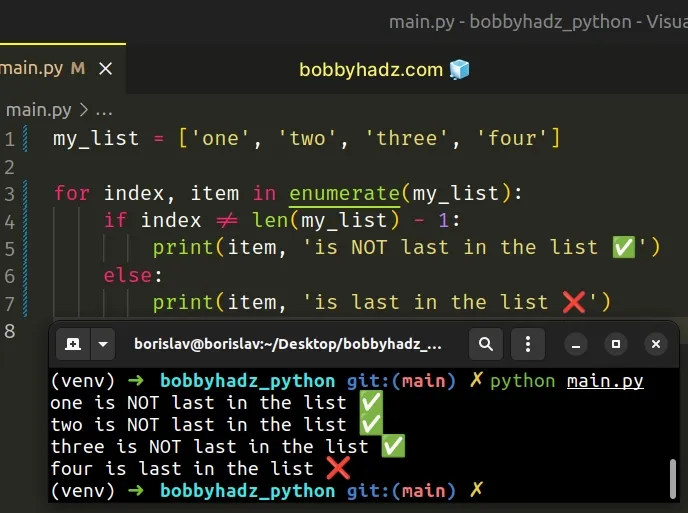Python indexes are zero-based, so the first index in a list is `0`, and the last index is `len(my_list) - 1`.

## #Checking if we are on the last iteration of the loop

If you need to check if the element is the last list item, change the not equals (!=) operator to the equals (==) operator.

main.py
```Copied!```my_list = ['one', 'two', 'three', 'four']

for index, item in enumerate(my_list):
if index == len(my_list) - 1:
print(item, 'is last in the list ✅')
else:
print(item, 'is NOT last in the list ❌')
``````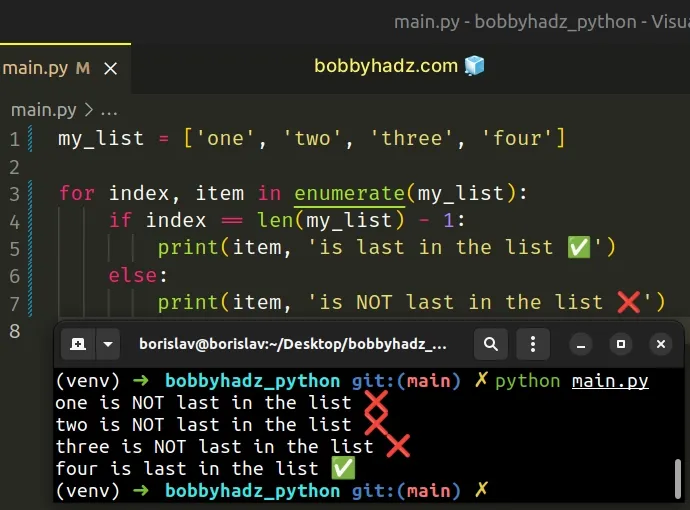The example checks if the current index is equal to the last index in the list.

## #Not performing an operation for the Last item in the List

If you don't want to perform an operation for the last item in the list, use a list slice that excludes it.

main.py
```Copied!```my_list = ['one', 'two', 'three', 'four']

for item in my_list[:-1]:
print(item, 'is NOT last in the list ✅')

print(my_list[-1], 'is last in the list ❌')
``````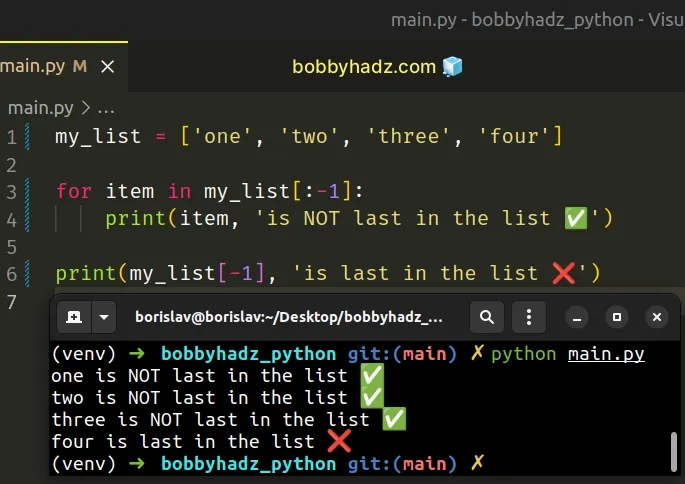The `my_list[:-1]` syntax returns a slice of the list that excludes the last element.

The syntax for list slicing is `my_list[start:stop:step]`.

The slice in the example starts at index `0` and goes up to, but not including the last item in the list.

Negative indices can be used to count backward, e.g. `my_list[-1]` returns the last item in the list and `my_list[-2]` returns the second-to-last item.

## #Joining without a separator after the last item

If you need to join the items in the list with a string separator, but don't want to add the separator after the last element, use the `str.join()` method.

main.py
```Copied!```my_list = ['one', 'two', 'three', 'four']

result_1 = '_'.join(my_list)
print(result_1)  # 👉️ 'one_two_three_four'

result_2 = ' '.join(my_list)
print(result_2)  # 👉️ 'one two three four'
``````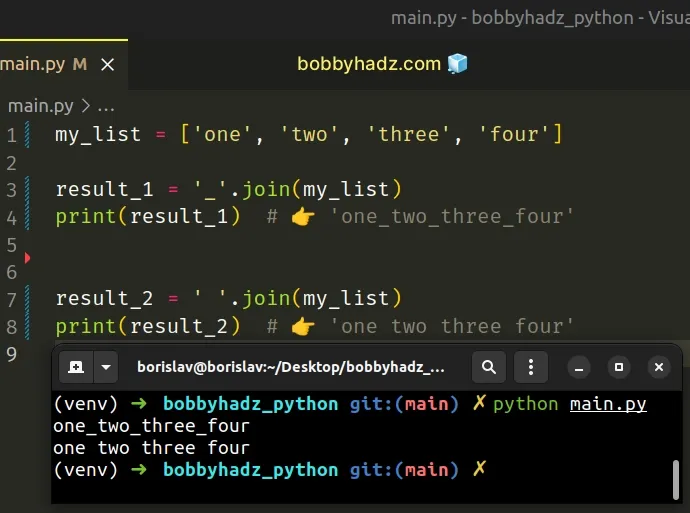The str.join method takes an iterable as an argument and returns a string which is the concatenation of the strings in the iterable.

Note that the method raises a `TypeError` if there are any non-string values in the iterable.

If your list contains numbers or other types, convert all of the values to string before calling `join()`.

main.py
```Copied!```my_list = ['one', 1, 'two', 2, 'three', 3]

list_of_strings = list(map(str, my_list))

result_1 = '_'.join(list_of_strings)
print(result_1)  # 👉️ 'one_1_two_2_three_3'

result_2 = ' '.join(list_of_strings)
print(result_2)  # 👉️ 'one 1 two 2 three 3'
``````

The string the method is called on is used as the separator between the elements.

If you don't need a separator and just want to join the iterable's elements into a string, call the `join()` method on an empty string.

main.py
```Copied!```my_list = ['one', 'two', 'three']

result_1 = ''.join(my_list)
print(result_1)  # 👉️ 'onetwothree'
``````